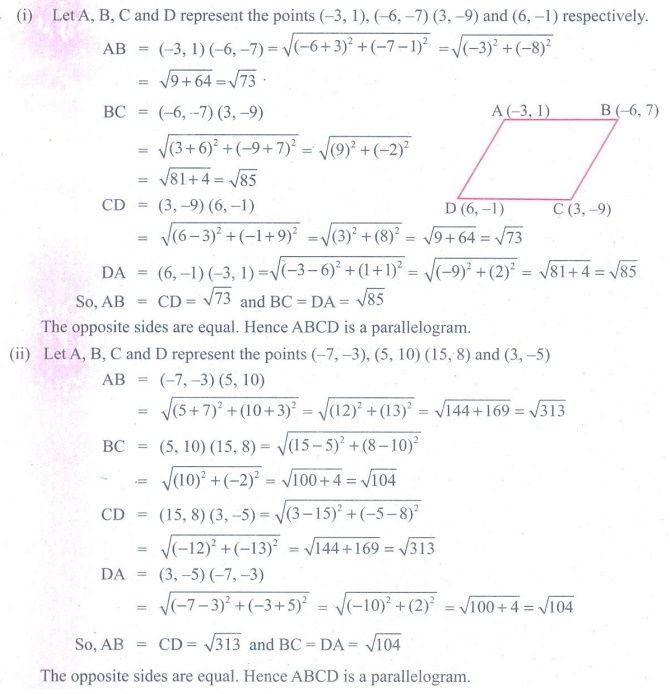Home | | Maths 9th std | Exercise 5.2: Distance between any Two Points

# Exercise 5.2: Distance between any Two Points

Maths : Coordinate Geometry: Book Back, Exercise, Example Numerical Question with Answers, Solution: Exercise 5.2: Distance between any Two Points

Coordinate Geometry: Distance between any Two Points

Exercise 5.2

1. Find the distance between the following pairs of points.

(i) (1, 2) and (4, 3)

(ii) (3,4) and (– 7, 2)

(ii) (a, b) and (c, b)

(iv) (3,– 9) and (–2, 3)2. Determine whether the given set of points in each case are collinear or not.

(i) (7,–2),(5,1),(3,4) (ii) (a,–2), (a,3), (a,0)3. Show that the following points taken in order form an isosceles triangle.

(i) A (5,4), B(2,0), C (–2,3) (ii) A (6,–4), B (–2, –4), C (2,10)4. Show that the following points taken in order form an equilateral triangle in each case.

(i) A(2, 2), B(–2, –2), C( -2√3 , 2√3 ) (ii) A(√3 , 2) , B (0,1), C(0,3)5. Show that the following points taken in order form the vertices of a parallelogram.

(i) A(–3, 1), B(–6, –7), C (3, –9), D(6, –1) (ii) A (–7, –3), B(5,10), C(15,8), D(3, –5)6. Verify that the following points taken in order form the vertices of a rhombus.

(i) A(3,–2), B (7,6),C (–1,2), D (–5, –6) (ii) A (1,1), B(2,1),C (2,2), D(1,2)7. A (–1, 1), B (1, 3) and C (3, a) are points and if AB = BC, then find ‘a’.8. The abscissa of a point A is equal to its ordinate, and its distance from the point B(1, 3) is 10 units, What are the coordinates of A?9. The point (x, y) is equidistant from the points (3,4)and(–5,6). Find a relation between x and y.10. Let A(2, 3) and B(2,–4) be two points. If P lies on the x-axis, such that AP = 3/7 AB, find the coordinates of P.11. Show that the point (11,2) is the centre of the circle passing through the points (1,2), (3,–4) and (5,–6)12. The radius of a circle with centre at origin is 30 units. Write the coordinates of the points where the circle intersects the axes. Find the distance between any two such points.Tags : Numerical Problems with Answers, Solution | Coordinate Geometry | Maths , 9th Maths : UNIT 5 : Coordinate Geometry
Study Material, Lecturing Notes, Assignment, Reference, Wiki description explanation, brief detail
9th Maths : UNIT 5 : Coordinate Geometry : Exercise 5.2: Distance between any Two Points | Numerical Problems with Answers, Solution | Coordinate Geometry | Maths